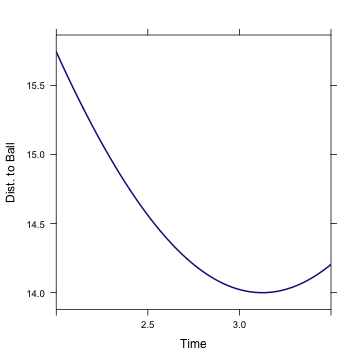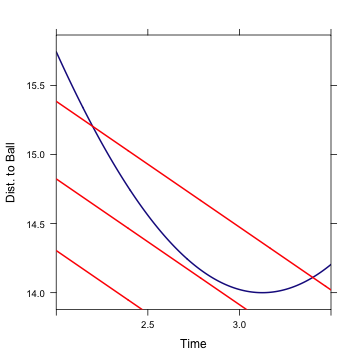# Dog Calculus is Really Economics

Tim Pennings has famously described his dog Elvis's fetching behavior
as consistent with calculus. Of course, Tim doesn't claim that even the dog of a mathematics professor can do calculus. So how is the dog finding a solution? If there's a method that works for a dog, perhaps the same method can work for a college student.

Here's a related problem. A student wants to earn enough money to pay her rent. She's working at a job that pays her at a certain rate, A. There's another job available, paying B. When should the student switch from job A to job B.

The answer is pretty obvious. Switch when $$A < B$$.

It's the same for Elvis. As Elvin runs along the beach, he's closing in on the ball. The dog runs at speed $$r$$. Let $$y$$ be Elvis's distance from the perpendicular point C. Then, as a function of time, Elvis's distance from the ball is
$d(t) = \sqrt{x^2 + (y_0-rt)^2}.$

Let's plot that out:

d = makeFun(sqrt(x^2 + (y0 - r * t)^2) ~ t, x = 14, r = 6.4, y0 = 20)
plotFun(d(t) ~ t, t.lim = range(2, 3.5), xlab = "Time", ylab = "Dist. to Ball")The graph shows that as Elvis runs down the beach, he gets closer to the ball. At first, running along the beach is a pretty good strategy and Elvis's closing velocity is fast. But then the closing velocity gets worse and worse. After running about three seconds, Elvis would run past the perpendicular point C. From then on, Elvis is getting farther away from the ball.

To keep running down the beach, away from the ball, is so silly that it's unremarkable that a dog doesn't do it. Instead, Elvis turns into the water at some point before reaching C. The trick is to know when to turn.

When Elvis does turn, he heads straight for the ball. This fact is unremarkable but it is very important. At any point, Elvis can head straight toward the ball by swimming. When he does so, his closing velocity on the ball will be exactly his swimming speed, $$s$$.The red lines on the graph show the distance to the ball when swimming. There are several red lines because Elvis might have choosen to jump into the water at any distance from it. But in every case, the lines have the same slope because, in every case, the closing velocity is $$s$$.

At the point where the slope of the red lines is steeper than the blue, it makes sense for Elvis to switch modes. He will close faster by swimming than by running.

How does Elvis know? He doesn't have to do a calculation other than these:
1. estimate the closing velocity as he's running
2. compare this closing velocity to his swimming speed

## Comparing to the Formula

“Somewhat surprisingly, the optimal path does not depend on $$z$$, as long as $$z$$ is larger than $$y$$.''

That this is a surprise is a result of the method of solution, not the problem. If you think about the problem as one of comparing closing velocities, it's obvious that the closing velocity far down the beach will be higher than the closing velocity near the run-to-swim transition point. The transition point is determined locally by comparing velocities and not globally by comparing total times of different possible routes.